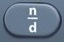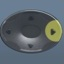# TemperatureBasics
Temperature is a measurement of hot or cold.

Temperature is measured in degrees, indicated by the ° sign.

There are two systems of temperature measurement:
(1) Fahrenheit, used in the USA, abbreviated as °F.
At 32 °F, water freezes.
At 212 °F, water boils.

(2) Celsius (also known as Centigrade), used in the rest of the world, abbreviated as °C.
At 0 °C, water freezes.
At 100 °C, water boils.

To convert °F to °C, the following formula is used:
°C = (°F – 32) ×$\bf\displaystyle\frac{5}{9}$

To convert °C to °F, the following formula is used:
°F = °C ×$\bf\displaystyle\frac{9}{5}$ + 32

When you encounter a temperature conversion question in the Science test, you will probably be given the appropriate temperature conversion formula.  You should also be allowed to use the calculator for such a question.

A temperature conversion question is an algebraic plug-in, where you plug in a number for a letter.

Question

The temperature outside is 50 °F.  What is the temperature in °C?

10 °C

See Plug-In.
°C = (°F – 32) ×$\bf\displaystyle\frac{5}{9}$

°C = (50 – 32) ×$\bf\displaystyle\frac{5}{9}$ = 10

Calculator Click What You See Commentblinker clears screen50-32×59(50-32)*$\bf\displaystyle\frac{5}{9}$ °C = (°F – 32) ×$\bf\displaystyle\frac{5}{9}$
Plug in 50 for °F.10 Answer
###### Temperature

Question

The temperature outside is 50 °C.  What is the temperature in °F?

122 °F

See Plug-In.
°F = °C ×$\bf\displaystyle\frac{9}{5}$ + 32

°F = 50 ×$\bf\displaystyle\frac{9}{5}$ + 32 = 122

Calculator Click What You See Commentblinker clears screen
50 ×95+ 32 50*$\bf\displaystyle\frac{9}{5}$+32 °F = °C ×$\bf\displaystyle\frac{9}{5}$ + 32
Plug in 50 for °C.122 Answer
###### Temperature

Practice – Questions

°C = (°F – 32) ×$\bf\displaystyle\frac{5}{9}$
1.  The temperature outside is 59 °F.  What is the temperature in °C?
A.  51
B.$\bf\displaystyle\frac{691}{5}$
C.  10
D.  15

°C = (°F – 32) ×$\bf\displaystyle\frac{5}{9}$
2.  The temperature outside is 68 °F.  What is the temperature in °C?
A.  2
B.$\bf\displaystyle\frac{772}{5}$
C.  20
D.  10

°F = °C ×$\bf\displaystyle\frac{9}{5}$ + 32
3.  The temperature outside is 60 °C.  What is the temperature in °F?
A.  41
B.$\bf\displaystyle\frac{140}{9}$
C.  10
D.  140

°F = °C ×$\bf\displaystyle\frac{9}{5}$ + 32
4.  The temperature outside is 30 °C.  What is the temperature in °F?
A.  86
B.  –$\bf\displaystyle\frac{10}{9}$
C.  68
D.  10

°F = °C ×$\bf\displaystyle\frac{9}{5}$ + 32
5.  The temperature outside is -30 °C.  What is the temperature in °F?
A.  10
B.  -30
C.  -22
D.  22